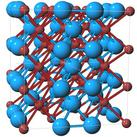Crystal Lattice Structures: Creation Date: 17 Feb 2007 Last Modified: 17 Feb 2007

# The V4Zn5 Crystal StructureYou can now

• Prototype: V4Zn5
• Pearson Symbol: tI18
• Strukturbericht Designation: none
• Space Group: I4/mmm (Cartesian and lattice coordinate listings available)
• Number: 139
• Other compounds with the structure: Pt8Ti
• Reference: Villars and Calvert, Pearson's Handbook Vol. IV, p. 5354.
• Primitive Vectors:  A1 = a X A2 = a Y A3 = ½ a X + ½ a Y + ½ c Z
• Basis Vectors:  B1 = 0 (Zn-I) (2a) B2 = + x1 A1 + x1 A2 = + x1 a X + x1 a Y (Zn-II) (8h) B3 = - x1 A1 - x1 A2 = - x1 a X - x1 a Y (Zn-II) (8h) B4 = + x1 A1 - x1 A2 = + x1 a X - x1 a Y (Zn-II) (8h) B5 = - x1 A1 + x1 A2 = - x1 a X + x1 a Y (Zn-II) (8h) B6 = + x2 A1 = + x2 a X (V) (8i) B7 = - x2 A1 = - x2 a X (V) (8i) B8 = + x2 A2 = + x2 a Y (V) (8i) B9 = - x2 A2 = - x2 a Y (V) (8i)

Go back to the body-centered cubic and related structures page.

Go back to Crystal Lattice Structure page.

 Structures indexed by: This is a mirror of an old page created at theNaval Research LaboratoryCenter for Computational Materials ScienceThe maintained successor is hosted at http://www.aflowlib.org/CrystalDatabase/ and published as M. Mehl et al., Comput. Mater. Sci. 136 (Supp.), S1-S828 (2017).